### SOLUTIONS TO INTEGRATION BY PARTS

SOLUTION 1 : Integrate. Let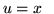and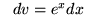so thatand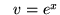.

Therefore,.

SOLUTION 2 : Integrate. Letand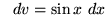so thatand.

Therefore,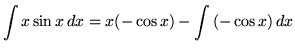.

SOLUTION 3 : Integrate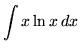. Letandso that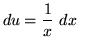and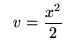.

Therefore,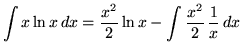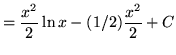.

SOLUTION 4 : Integrate. Letand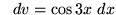so thatand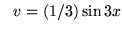.

Therefore,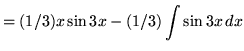.

SOLUTION 5 : Integrate. Let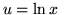and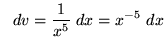so thatand.

Therefore,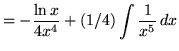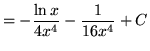.

SOLUTION 6 : Integrate. Let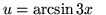andso that (Don't forget to use the chain rule when differentiating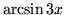.)and.

Therefore,.

Now use u-substitution. Letso that,

or.

Then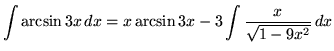+ C+ C+ C .

SOLUTION 7 : Integrate. Letandso thatand.

Therefore,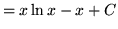.

SOLUTION 8 : Integrate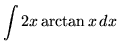. Letandso thatand.

Therefore,(Addin the numerator. This will replicate the denominator and allow us to split the function into two parts.)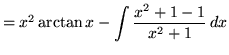(Please note that there is a TYPO in the next step. The second "-" sign should be a "+" sign. However, subsequent steps are correct.)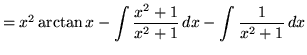(The remaining steps are all correct.).

SOLUTION 9 : Integrate. Letandso thatand.

Therefore,.

Integrate by parts again. Letandso thatand.

Hence,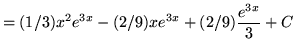.